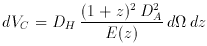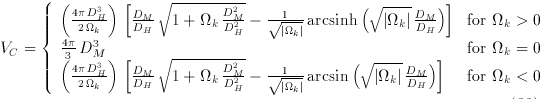### 9. COMOVING VOLUME

The comoving volume VC is the volume measure in which number densities of non-evolving objects locked into Hubble flow are constant with redshift. It is the proper volume times three factors of the relative scale factor now to then, or (1 + z)3. Since the derivative of comoving distance with redshift is 1 / E (z) (defined above), the angular diameter distance converts a solid angle dinto a proper area, and two factors of (1 + z) convert a proper area into a comoving area, the comoving volume element in solid angle dand redshift interval dz is(27)

where DA is the angular diameter distance at redshift z and E (z) is defined above (Weinberg 1972, p. 486; Peebles 1993, pp. 331-333). The comoving volume element is plotted in Figure 5. The integral of the comoving volume element from the present to redshift z gives the total comoving volume, all-sky, out to redshift z(28)Figure 5. The dimensionless comoving volume element (1 / DH)3 (dVC / dz). The three curves are for the three world models, (M,) = (1, 0), solid; (0.05, 0), dotted; and (0.2, 0.8), dashed.

(Carrol, Press & Turner 1992), where DH3 is sometimes called the Hubble volume. The comoving volume element and its integral are both used frequently in predicting number counts or luminosity densities.